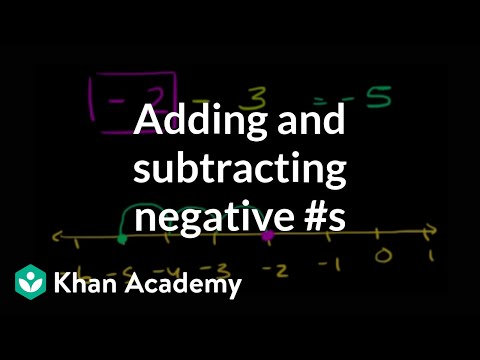Video

# Negative number word problem: Alaska (Full video)

Description: In this video, we figure out the temperature in Fairbanks, Alaska by adding and subtracting negative numbers. Amanda notices the temperature at 5pm is negative nine degrees Celsius. Alright, so at 5pm we end up at negative nine degrees Celsius, so let's just say this is zero, negative one, two, three, four, five, six, seven, eight, nine. So we end up at negative nine degrees Celsius.

### Other videos you might be interested in### Absolute value as distance between numbers (Full video)